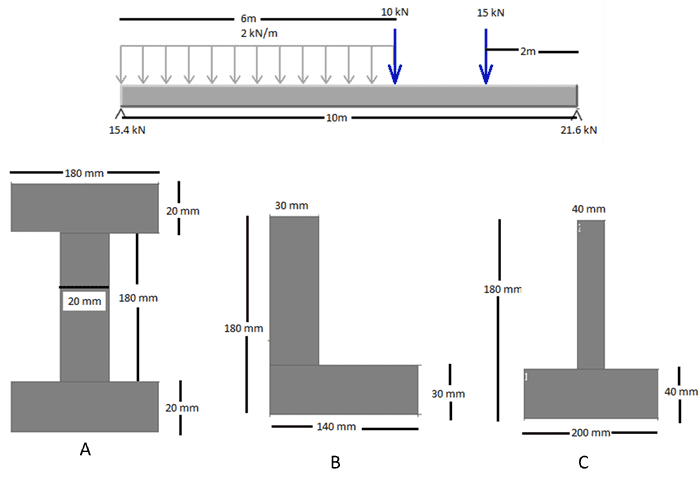# Maximum stress in beams with different cross sections

## Homework Statement

Here is the problem:I have to calculate the maximum stress for A, B and C.

## Homework Equations

I used:

σ = y*F*L / 4*I (but I don't think this is right)

σ = maximum stress
y = Perpendicular distance from to neutral axis
L = length of beam
I = moment of Inertia

## The Attempt at a Solution

Using:
σ = y*F*L / 4*I

I got the following:
A = 1.241
B = 5.562
C = 4.085